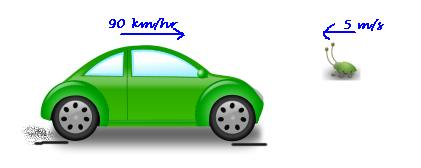## Force and Laws of Motion

(MCQs)

Q1: An object moving at constant velocity in an inertial frame must:

(a) have a net force acting on it
(b) have zero net force acting on it
(c) not have any force of gravity on it
(d) stop after some time due to gravity

Q2: When a bus starts suddenly from rest, the passengers sitting inside the bus move backwards.  This illustrates an example of

(a) Newton First Law of Motion
(b) Newton Second Law of Motion
(c) Newtons Third Law of Motion
(d) None of these

Q3: One newton is the force ____________

(a) of gravity on a 1 kg body
(b) of gravity on a 1 g body
(c) which gives a 1 g body an acceleration of 1 cm/s2
(d) which gives a 1 kg body an acceleration of 1m/s2

Q4: The property of inertia is the largest in

(a) a toy car
(b) a motocycle
(c) a car
(d) a truck

Q5: A force of 5N acts on a body. What is the acceleration produced in m/s2, if the weight of the body is 9.8N.

(a) 5
(b) 0.5
(c) 1.96
(d) 49

Q6: Acceleration acts always in the direction __________
(a) of the displacement
(b) of the initial velocity
(c) of the net force
(d) of the final velocity

Q7: The acceleration produced in a body by an acting force of given magnitude depends upon _____.

(a) size of the body
(b) mass of the body
(c) shape of the body
(d) all of these

Q8: A ball is moving in a circle at fixed speed. Which of the following statement is TRUE?

(a) There is no acceleration present.
(b) There must be only one force acting on it.
(c) Acceleration is present but has constant magnitude.
(d) Acceleration  is present and acts tangent to the circle.

Q9: Action and reaction act on

(a) same body but in opposite directions
(b) same body and in same direction
(c) different bodies but in opposite directions
(d) different bodies but in same direction

Q10: A body of weight W is suspended from the ceiling of a room through a rope of weight R. The ceiling pulls the rope by a force of

(a) W
(b) R
(c) W + R
(d) (W+R)/2

1: (b) have zero net force acting on it
2: (a) Newton First Law of Motion
3: (d) which gives a 1 kg body an acceleration of 1m/s2
4: (d) a truck
5: (a) 5
6: (c) of the net force
7: (b) mass of the body
8: (c) Acceleration is present but has constant magnitude.
9: (c) different bodies but in opposite directions
10:  (c) W + R1.Good questions...

2.3.Best... Website for students

4.Best... Website for students

1.5.6.Only 10 bits is not enough.our sir told to write 100 bits

7.8.SuperbbSuperbbb

9.10.11.12.